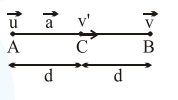# An engine of a train, moving with uniform acceleration,Question:

An engine of a train, moving with uniform acceleration, passes the signal-post with velocity $u$ and the last compartment with velocity v. The velocity with which middle point of the train passes the signal post is:

1. $\sqrt{\frac{\mathrm{v}^{2}+\mathrm{u}^{2}}{2}}$

2. $\frac{\mathrm{v}-\mathrm{u}}{2}$

3. $\frac{u+v}{2}$

4. $\sqrt{\frac{\mathrm{v}^{2}-\mathrm{u}^{2}}{2}}$

Correct Option: 1

Solution:$\left(\mathrm{v}^{\prime}\right)^{2}=\mathrm{u}^{2}+2 \mathrm{ad}$'

$v^{2}=\left(v^{\prime}\right)^{2}+2 a d$

solving, we get

$\mathrm{v}^{\prime}=\sqrt{\frac{\mathrm{v}^{2}+\mathrm{u}^{2}}{2}}$